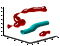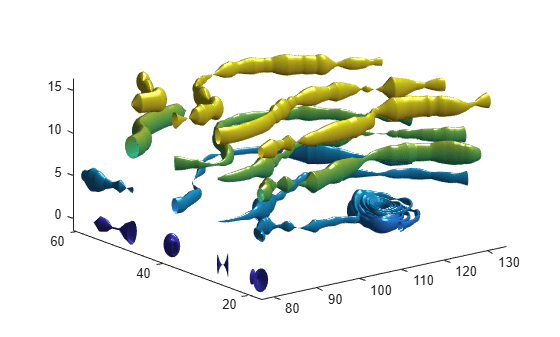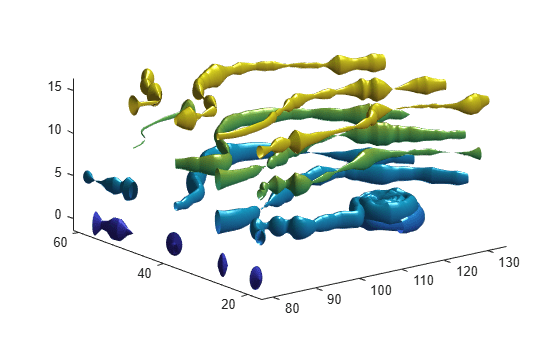streamtube

Create 3-D stream tube plotSyntax

streamtube(X,Y,Z,U,V,W,startx,starty,startz)
streamtube(U,V,W,startx,starty,startz)
streamtube(vertices,X,Y,Z,divergence)
streamtube(vertices,divergence)
streamtube(vertices,width)
streamtube(vertices)
streamtube(...,[scale n])
streamtube(ax,...)
h = streamtube(...)

Description

streamtube(X,Y,Z,U,V,W,startx,starty,startz) draws stream tubes from vector volume data U, V, W.

The arrays X, Y, and Z, which define the coordinates for U, V, and W, must be monotonic, but do not need to be uniformly spaced. X, Y, and Z must have the same number of elements, as if produced by meshgrid.

startx, starty, and startz define the starting positions of the streamlines at the center of the tubes.

The width of the tubes is proportional to the normalized divergence of the vector field.

streamtube(U,V,W,startx,starty,startz) assumes X, Y, and Z are determined by the expression

[X,Y,Z] = meshgrid(1:n,1:m,1:p)

where [m,n,p] = size(U).

streamtube(vertices,X,Y,Z,divergence) assumes precomputed streamline vertices and divergence. vertices is a cell array of streamline vertices (as produced by stream3). X, Y, Z, and divergence are 3-D arrays.

streamtube(vertices,divergence) assumes X, Y, and Z are determined by the expression

[X,Y,Z] = meshgrid(1:n,1:m,1:p)

where [m,n,p] = size(divergence).

streamtube(vertices,width) specifies the width of the tubes in the cell array of vectors, width. The size of each corresponding element of vertices and width must be equal. width can also be a scalar, specifying a single value for the width of all stream tubes.

streamtube(vertices) selects the width automatically.

streamtube(...,[scale n]) scales the width of the tubes by scale. The default is scale = 1. When the stream tubes are created, using start points or divergence, specifying scale = 0 suppresses automatic scaling. n is the number of points along the circumference of the tube. The default is n = 20.

streamtube(ax,...) plots into the axes object ax instead of into the current axes object (gca).

h = streamtube(...) returns a vector of surface objects (one per start point) used to draw the stream tubes.

Examples

collapse all

Use the streamtube function to indicate flow in the wind data set. The inputs include the coordinates, vector field components, and starting location for the stream tubes.

[sx,sy,sz] = meshgrid(80,20:10:50,0:5:15);
streamtube(x,y,z,u,v,w,sx,sy,sz);
view(3);
axis tight
camlight;
lighting gouraudUse vertex data returned by the stream3 function and divergence data to visualize flow.

[sx,sy,sz] = meshgrid(80,20:10:50,0:5:15);
verts = stream3(x,y,z,u,v,w,sx,sy,sz);
div = divergence(x,y,z,u,v,w);
streamtube(verts,x,y,z,-div);
view(3);
axis tight